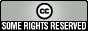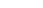## Differential Expression Analysis with Bioconductor and Python

#### Sunday, September 13, 2009 at 10:22 PM EDT

This post demonstrates performing differential expression analysis of short read sequencing data using a combination of Python and the R statistical language. Python is used a glue language to manipulate and prepare count data from short read sequencing. R and the Bioconductor package are used to perform the statistical analysis. The excellent rpy2 package connection Python and R.

Scientists often need to compare expression results from multiple experimental conditions. This can be done with microarray analysis and the wide variety of associated statistical tests, but many researchers are now utilizing short read sequencing. Experiments identify transcript prevalence through digital gene expression under multiple conditions, and we are interested in extracting statistically meaningful differences from the results. Similarly, we may be interested in differences resulting from short RNA discovery or other next generation sequencing applications.

Short read differential expression analysis differs from microarray analyses as it is based on counts instead of intensity scores. The edgeR package in Bioconductor fits count data to a statistical model considering sample to sample variation, and performs Fisherâ€™s exact test to provide p-values associated with changes in expression between samples.

Here we will consider the case of a two experiment analysis. A simple Example CSV file has counts for 5 different genes under 2 conditions and total count information for the full experiment. We read the file into a dictionary of counts keyed by each condition and the gene name:

```
import csv
import collections

"""Read count information from a simple CSV file into a
dictionary.
"""
counts = collections.defaultdict(dict)
with open(in_file) as in_handle:
for parts in reader:
region_name = parts
region_counts = [float(x) for x in parts[1:]]
for ci, condition in enumerate(conditions):
counts[condition][region_name] = region_counts[ci]
return dict(counts)
```

The dictionary is organized into NumPy matrices; NumPy is a powerful numerical package for Python which integrates smoothly with our rpy2 interface to import directly into R. Here we organize our conditions and genes and then push the count data into a matrix where the columns are conditions and the rows are genes; each item is a count value. This is returned along with associated data:

• groups â€” A list of experimental groups, which can be used to analyze replicates. Here, two groups with a single replicate each are defined.
• sizes â€” The total number of counts for each experiment. This is extracted from the â€œTotalâ€ row of the CSV count file, and will be used for normalization of during the statistical analysis.

```
import numpy

def get_conditions_and_genes(work_counts):
conditions = work_counts.keys()
conditions.sort()
all_genes = []
for c in conditions:
all_genes.extend(work_counts[c].keys())
all_genes = list(set(all_genes))
all_genes.sort()
sizes = [work_counts[c]["Total"] for c in conditions]
all_genes.remove("Total")
return conditions, all_genes, sizes

def edger_matrices(work_counts):
conditions, all_genes, sizes = get_conditions_and_genes(work_counts)
assert len(sizes) == 2
groups = [1, 2]
data = []
final_genes = []
for g in all_genes:
cur_row = [int(work_counts[c][g]) for c in conditions]
if sum(cur_row) > 0:
data.append(cur_row)
final_genes.append(g)
return (numpy.array(data), numpy.array(groups), numpy.array(sizes),
conditions, final_genes)
```

The organized data is now ready to be pushed directly into an edgeR Bioconductor analysis using the rpy2 interface. Three R functions are called: the data matrices are organized into a `DGEList` object, this object is passed to `deDGE` which does the actual digital gene expression analysis, and finally `topTags` is called to retrieve a vector of differential expression p-values. The vector is translated into a Python object from which we extract the p-values and re-organize them by the initial gene indexes. The ordered p-values are then returned.

```
import rpy2.robjects as robjects
import rpy2.robjects.numpy2ri

def run_edger(data, groups, sizes, genes):
robjects.r('''
library(edgeR)
''')
params = {'group' : groups, 'lib.size' : sizes}
dgelist = robjects.r.DGEList(data, **params)
ms = robjects.r.deDGE(dgelist, doPoisson=True)
tags = robjects.r.topTags(ms, pair=groups, n=len(genes))
indexes = [int(t) - 1 for t in tags.rownames()]
pvals = list(tags[-2])
assert len(indexes) == len(pvals)
pvals_w_index = zip(indexes, pvals)
pvals_w_index.sort()
assert len(pvals_w_index) == len(indexes)
return [p for i,p in pvals_w_index]
```

The final results are written to a CSV file with our ordered genes, conditions and p-value probabilities. Each gene is associated with the initial count data and p-values, the genes are sorted by p-value with the most differentially expressed genes placed first, and the ordered information is written out:

```
def write_outfile(outfile, genes, conditions, work_counts, probs):
with open(outfile, "w") as out_handle:
writer = csv.writer(out_handle)
writer.writerow(["Region"] +
["%s count" % c for c in conditions] + ["edgeR
p-value"])
out_info = []
for i, gene in enumerate(genes):
counts = [int(work_counts[c][gene]) for c in conditions]
out_info.append((probs[i], [gene] + counts))
out_info.sort()
[writer.writerow(start + [prob]) for prob, start in out_info]
```

Our example output file shows the results. The full script is available for you to use and customize for your own analyses. This example demonstrates the power of the rpy2 interface. Input and output data is happily manipulated in a familiar language such as Python, and can be seamlessly integrated with excellent statistical computation in Bioconductor and other specialized R packages.